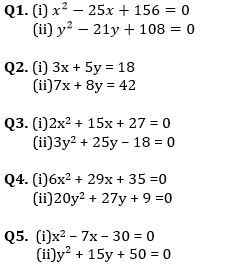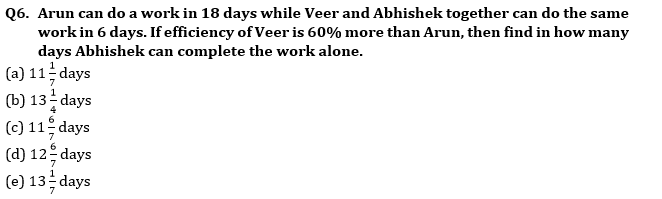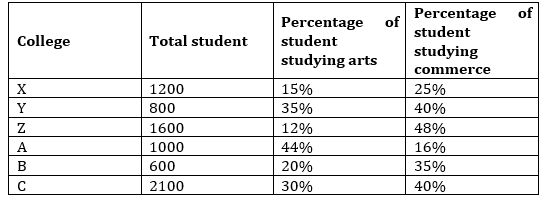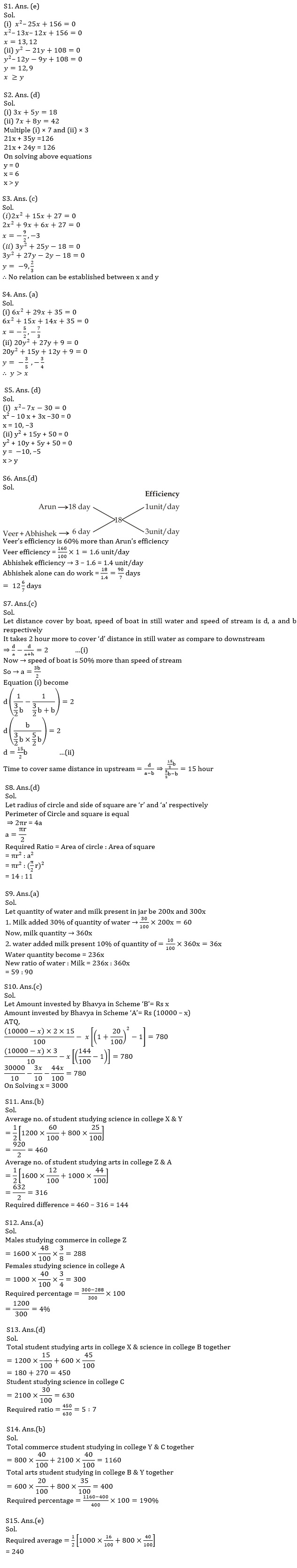Latest Banking jobs   »   Quantitative Aptitude Quiz For IBPS RRB...

# Quantitative Aptitude Quiz For IBPS RRB PO, Clerk Prelims 2021- 24th July

(a) x < y
(b) x ≤ y
(c) x = y or no relation can be established between x and y.
(d) x > y
(e) x ≥ yQ7. A boat covers a certain distance in lake whose speed of water current is zero and takes 2 hours more than the time taken to cover same distance downstream in the river. If speed of boat is 50 % more than the speed of stream of river then, find the time taken by boat to cover the same distance upstream (consider speed of boat in still water in lake and river is same) ?
(a) 10 hours
(b) 12 hours
(c) 15 hours
(d) 15/2 hours
(e) 13 hours

Q8. Find the ratio of area of a circle to area of square if perimeter of circle and square is equal?
(a) 11 : 14
(b) 11 : 4
(c) π : 11
(d) 14 : 11
(e) 2 : π

Q9. A Jar contain water and milk in the ratio of 2: 3. Some milk is added in the Jar whose amount is equal to 30% of water present in the Jar. After this some water is added whose amount is equal to 10% of quantity of milk present in Jar presently. What is the new ratio of water and milk in the Jar.
(a) 59 : 90
(b) 11 : 18
(c) 90 : 59
(d) 18 : 11
(e) 57 : 67

Q10. Bhavya have 10,000 Rs. He invested some amount in Scheme ‘A’ which offers 15% p.a. at SI and rest in Scheme ‘B’ which offers 20% p.a. at CI. Interest earned form scheme ‘A’ after 2 years is 780Rs more than interest earned form scheme ‘B’ after 2 years. Find the amount invested in Scheme ‘B’?
(a) 8000 Rs
(b) 7000 Rs
(c) 3000 Rs
(d) 2000 Rs
(e) 5000 Rs

Direction (11-15): Study the table carefully and answer the following questions.
Table given below shows the total no. of student in six different college and percentage of student studying arts and commerce.
Total student in each college = Student studying arts, commerce & science.Q11. What is the difference between average of no. of student studying science in college X and Y and average of no. of student studying arts in college Z and A?
(a) 244
(b) 144
(c) 164
(d) 182
(e) 128

Q12. If ratio of number of males to number of females studying commerce in college Z & studying science in college A is 3 : 5 and 1 : 3 respectively then number of males studying commerce in college Z is what percent more/less than number of females studying science in college A?
(a) 4%
(b) 24%
(c) 12%
(d) 8%
(e) 16%

Q13. What is ratio of total number of students studying arts in college X and number of students studying science in college B together to number of students studying science in college C?
(a) 2 : 5
(b) 4 : 7
(c) 3 : 5
(d) 5 : 7
(e) 2 : 3

Q14. Total number of commerce students studying in college Y and C together is what percent more/less than total number of arts students studying in college B and Y together?
(a) 130%
(b) 190%
(c) 210%
(d) 165%
(e) 235%

Q15. What is the average of number of students studying commerce in college A and in college Y ?
(a) 160
(b) 180
(c) 210
(d) 260
(e) 240

Solutions